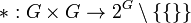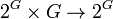# Hypergroup

This is a variation of group|Find other variations of group | Read a survey article on varying group
QUICK PHRASES: variation of group where the multiplication operation is multi-valued
NOTE: This page is about the abstract algebraic notion of hypergroup. There is a related notion of hypergroup that comes up in probability theory and measure theory, which is at hypergroup (measure theory).

## Definition

A hypergroup is a set$G$ equipped with a binary operation$*: G \times G \to 2^G \setminus \{ \{ \} \}$, i.e., a multi-valued binary operation, satisfying some conditions. The right side denotes the power set of$G$ minus the empty subset of$G$, because the binary operation is required to give at least one output for every input pair.

Prior to stating the condition, we note that$*$ can be extended to an operation$2^G \times 2^G \to 2^G$ given by$X * Y = \bigcup_{x \in X, y \in Y} (x * y)$. Similarly, we can extend$*$ to operations$2^G \times G \to 2^G$ and$G \times 2^G \to 2^G$.

Condition name What it means Explanation
multi-valued version of associativity For any$a,b,c \in G$,$(a * b) * c = a * (b * c)$ as sets. Left side: Note that$a * b$ is a subset, say$S$, of$G$.$(a * b) * c = S * c$ is defined as the union$\bigcup_{s \in S} s * c$.
Right side: Suppose$T = b * c$. Then,$a * (b * c) = a * T = \bigcup_{t \in T} (a * t)$.
multi-valued version of quasigroup type condition For any$a \in G$,$a * G = G * a = G$.

Note that if the operation is single-valued and the underlying set of$G$ is non-empty, then$G$ becomes a group under$*$. This follows from the proof of associative quasigroup implies group (our statement is actually a little more general than that statement, because we are not assuming unique solutions to equations, but the proof does not use uniqueness).

## Examples

We can take the quotient of a hypergroup by any equivalence relation and get a hypergroup structure on the quotient set. Since groups are hypergroups to begin with, quotients of groups by various sorts of equivalence relations give hypergroups. Some related examples are: